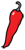The Grand Locus / Life for statistical sciences

## The fallacy of (in)dependenceIn the post Why p-values are crap I argued that independence is a key assumption of statistical testing and that it almost never holds in practical cases, explaining how p-values can be insanely low even in the absence of effect. However, I did not explain how to test independence. As a matter of fact I did not even define independence because the concept is much more complex than it seems.

Apart from the singular case of Bayes theorem, which I referred to in my previous post, the many conflicts of probability theory have been settled by axiomatization. Instead of saying what probabilities are, the current definition says what properties they have. Likewise, independence is defined axiomatically by saying that events $A$ and $B$ are independent if $P(A \cap B) = P(A)P(B)$, or in English, if the probability of observing both is the product of their individual probabilities. Not very intuitive, but if we recall that $P(A|B) = P(A \cap B)/P(B)$, we see that an alternative formulation of the independence of $A$ and $B$ is $P(A | B) = P(A)$. In other words, if $A$ and $B$ are independent, observing $B$ does not change the probability of observing $A$.

So, to know whether $A$ and $B$ are independent we just need to compare $P(A)$ and $P(A | B)$... right? In theory yes, but in practice, those probabilities are unknown. Most of the time, they are even undefined, which has far-reaching implications. To do statistics you don't need a brain, you just need a computer, and nowadays many people have a computer. Whence those countless statistical claims made without any care for the underlying probability.

For example, being vaccinated dramatically increases you chances of winning the lottery. Obviously vaccination has nothing to do with the lottery process. However, if we consider the worldwide population, vaccinated people more often live in the Western world and are more likely to play (and win) the lottery. There is an ambiguity on what P stands for. Is it choosing the lottery numbers at random or choosing a person in the human population at random? This last example, however naive, illustrates that the exact same events A and B can be sampled under distinct probabilistic frameworks. They can be independent under a sampling scheme and dependent under another one.

It is tempting to conclude that statistically dependent events are causally related, but the last example shows one of the many pitfalls of this approach. The question of causality has been haunting statisticians for decades but never adequately addressed. There is a general confusion between the occurrence of an event and its sampling. There is no reason why sampling, even independent, should follow the occurrence of an event — in the previous example, sampling of the lottery results, the human population, of the population of people who play the lottery are all reasonable. Let me summarize this in what I will call the fallacy of statistical dependence:

Causality is a relationship between the occurrence of events. Statistical dependence is a relationship between their sampling.

Great! Statistical dependence and causality are orthogonal concepts, so why should we care for independence at all? Because it is intimately connected to information, which I will explain further in the next post.